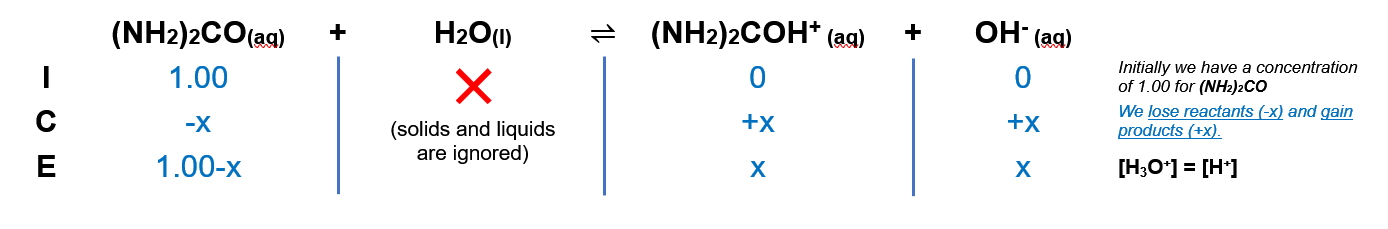# Problem: The pH of a 1.00 M solution of urea, a weak organic base, is 7.050.Calculate the Ka of protonated urea.

###### FREE Expert Solution

We’re being asked to calculate the Ka of protonated urea (The pH of 1.00 M urea, a weak organic base is 7.050).

Urea ((NH2)2CO) is a weak base.

Remember that weak bases partially dissociate in water and that bases accept H+ from the acid (water in this case).

The dissociation of Urea is as follows:

(NH2)2CO (aq) + H2O(l)  (NH2)2COH+ (aq) + OH-(aq)

From this, we can construct an ICE table.

Remember that liquids are ignored in the ICE table.Kb expression for (NH2)2CO  is:

Liquids are ignored in the Kb expression.

Note that each concentration is raised by the stoichiometric coefficient: [(NH2)2CO], [OH] and [(NH2)2COH+] are raised to 1.

98% (176 ratings)###### Problem Details

The pH of a 1.00 M solution of urea, a weak organic base, is 7.050.

Calculate the Ka of protonated urea.